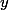# Martin's Blog

## Hensel's lemma and algebraic functions

Posted by Martin Orr on Monday, 05 April 2010 at 22:34

An algebraic function is a function which we obtain by solving a polynomial in two variables `` and `` to write `` as a function of ``. In general polynomials have more than one root, so (informally) we get a multi-valued function. In this post I will restrict attention to regions of the plane in which we can unambiguously pick a single "branch" of the function. What happens where branches meet will be the subject of a later post.

I shall give an algorithm for expressing an algebraic function (in such a nicely behaved region) as a power series, thereby proving that a power series solution to the original polynomial exists. The generalisation of this result to a complete discrete valuation ring is Hensel's lemma, and is particularly important to number theorists in the case of p-adic integers (which were invented by Hensel). In this post I will focus on the case of algebraic functions, as it is easier to apply geometric intuition.

Read more...

1 2 3 4 5 Next »